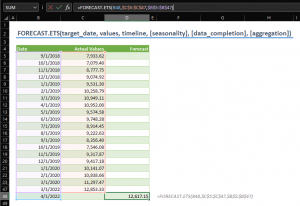The FORECAST.ETS function is a Statistical function that predicts a future value by using existing values with a seasonal pattern. The function is using the AAA version (additive error, additive trend, and additive seasonality) of the Exponential Smoothing (ETS) algorithm. You can use the forecasting function to predict future sales, inventory requirements, or consumer trends. In this guide, we’re going to show you how to use the FORECAST ETS function and go over some tips and error handling methods.

## Supported Versions

• Not supported Excel for the Web, iOS, or Android.

## FORECAST.ETS Function Syntax

FORECAST.ETS(target_date, values, timeline, [seasonality], [data_completion], [aggregation])

## Arguments

 target_date The data point to use to predict a value. Target date should be chronologically after than the end of the historical timeline. values The historical values in data points. (y values) timeline The historical data points (dates). (x values) [seasonality] Optional. A positive numeric value represents the periods in a season. 0: No seasonality, linear prediction. 1: Automatic. Default value. [data_completion] Optional. This determines how Excel treats missing data. 0: Treat as zero (0). 1: The average of the neighboring points. Default values. [aggregation] Optional. The aggregation method for the values with the same timestamp. 1: AVERAGE (Default) 2: COUNT 3: COUNTA 4: MAX 5: MEDIAN 6: MIN 7: SUM

## FORECAST.ETS Function Examples

### Default form

The FORECAST.ETS function needs the date for the data point you want to forecast (target_date) and the historical data (values, timeline) to work in its default state. The remaining three arguments are optional.

FORECAST.ETS(target_date, values, timeline)

• target_date: B48
• values: C5:C47
• timeline: B5:B47In this form, Excel calculates the seasonality automatically. If there are empty values, Excel fills them accordingly to the average of the neighboring points. Finally, if there are multiple values on the same data point (date), Excel aggregates them by calculating the average of those. You can alter each of these assumptions by populating the optional arguments.

### Charting

A single forecasted value will not tell you much. The best way to review and compare the forecasted data is to forecast for more points and use a line chart to see the trend. All you need to do is to generate more data points and copy the formula. Just pay attention to absolute and relative references, since you will want to keep ranges of the historical data.

In the following example you can see a chart that is generated from the formula with optional arguments. We used named ranges to refer to the optional argument cells in the cells G5, G6 and G7.Note that, cell D48 has the same value as the last historical data just to connect lines in the graph.

### Optional arguments

Using optional arguments is related to the dataset and the data origin. If you are in doubt, do not hesitate to try a different combination to find proper options for your data. Here is an example of how different options can alter the predicted values.## Remarks

• If the target_date is chronologically before the end of the historical timeline, FORECAST.ETS returns the #NUM! error.
• The date values in the timeline range aren't required to be sorted. However, if a constant step can't be identified in the provided timeline, the function returns #NUM!
• If the timeline range contains duplicate values, FORECAST.ETS will return the #VALUE! error.
• If the ranges of the timeline and values aren't of the same size, the function will return the #N/A
• The maximum value for the seasonality is 8760 (number of hours in a year). Any number above will return #NUM!

## Excel Forecasting Functions

 Forecasting with linear regression FORECAST.LINEAR Seasonality in forecasting using Exponential Triple Smoothing FORECAST.ETS.SEASONALITY Confidence interval in forecasting using Exponential Triple Smoothing FORECAST.ETS.CONFINT Statistical values in forecasting using Exponential Triple Smoothing FORECAST.ETS.STAT